# Category Mathematics Class -12

## Differential Equations Class 12 notes | IIT-JEE study material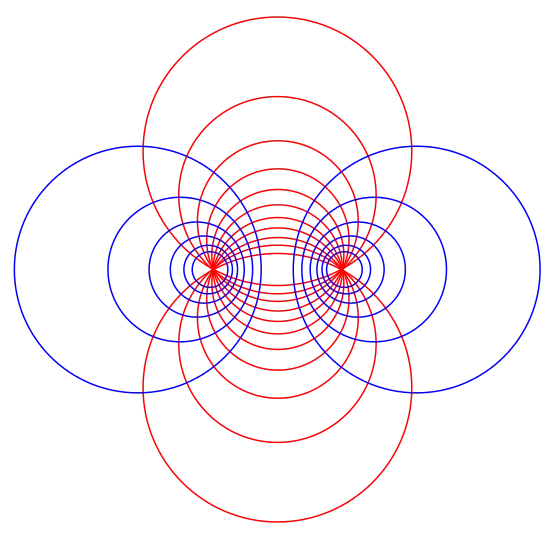So, in calculus we are starting the topic of differential equations, we will be seeing what does a differential equation exactly means, what are partial differential equations, the significance of differential equations in various disciplines is very vast, we will…

## Applications of Derivatives class 12 revision notes | IIT-JEE notes, study material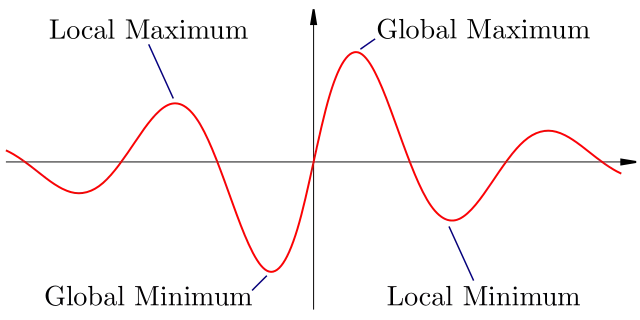We have analyzed the concept of derivative/Differentiation of a function, this concept is widely used in various fields like math, physics, engineering sciences, etc, derivatives are used to analyze any function by calculating its first and second derivative, Hence we…

## Methods of Differentiation | Concept Booster IIT-JEE Class 12 notes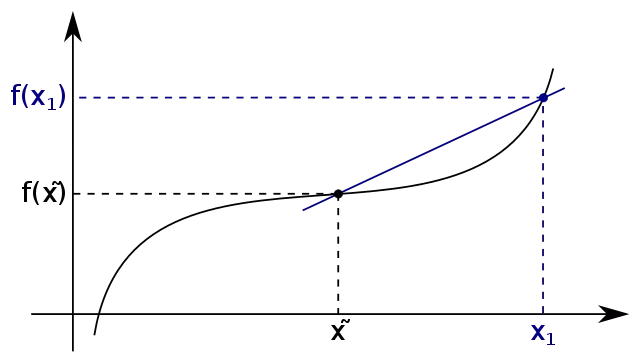Methods of Differentiation Here we will learn methods of Differentiation, We had already studied the two meanings of derivative(differentiation) of a function which is the geometrical and physical meanings. Since we know what does the differentiation of a function means…

## Differentiability Class 12 | IIT JEE Notes, Concept BoosterDifferentiability The concept of the differentiability (derivability) of a function tells us about whether a given function will be differentiable or not. If the function is differentiable then we will use Methods of differentiation to differentiate a function. Actually, this…

## Functions | Concept Booster, IIT JEE, Class 12 notesKnow Functions, Types of Functions, What is Composite Function ? Periodic Functions ? Properties of functions. You should learn set theory and relations before learning about functions. Functions A function defined from set A to set B is a special…

## Three Dimensional Geometry (part – 2) , The Planes | study material for IIT JEE | concept booster, chapter highlights/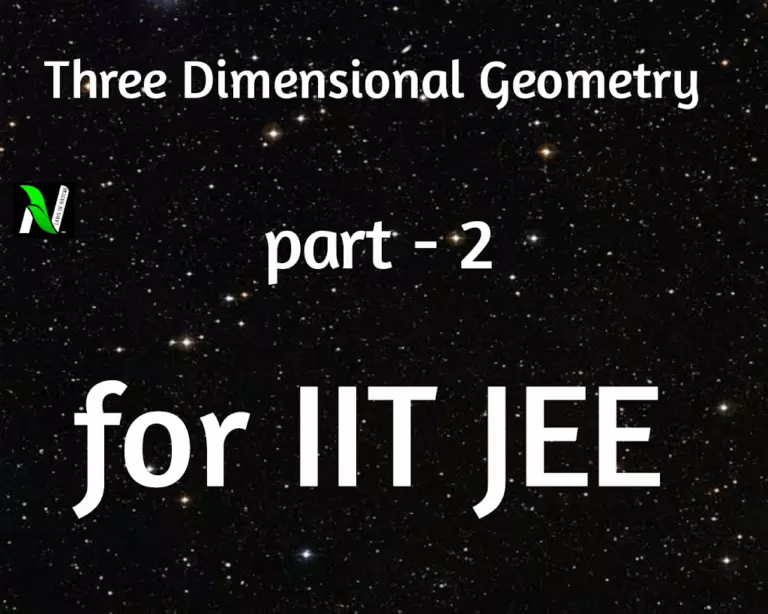THREE DIMENSIONAL GEOMETRY ( PART – 2), THE PLANES THE PLANES Plane is the surface such that if any two points are taken on it, then the line joining the two points lies on it. General equation of plane is…

## Three dimensional geometry (part-1), Lines | study material for IIT JEE | concept booster , chapter highlights.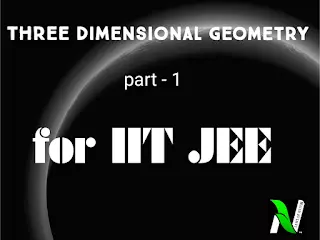THREE DIMENSIONAL GEOMETRY ORIGIN In the following diagram X’OX , Y’OY and Z’OZ are three mutually perpendicular lines , which intersect at point O. Then the point O is called origin. COORDINATE AXES In the above diagram X’OX is called…

## Inverse trigonometrc function IIT JEE study material | ITF concept booster | ITF notes | ITF important formulae.

INVERSE TRIGONOMETRY FUNCTION INVERSE FUNCTION If f:X⟶ Y is a function which is both one – one and onto, then it’s inverse function f^-1:Y⟶X is defined as: y = f(x) ⟺ f^-1(y)=x Such that , ∀x∈X , ∀y∈Y INVERSE TRIGONOMETRY…

## Properties and solutions of triangle for IIT-JEE (mains & advanced) | chapter notes | study material class 12.PROPERTIES AND SOLUTIONS OF TRIANGLE Here, there are some key concepts regarding the properties and solutions of triangles. these are very important for the students who preparing for IIT-JEE and other equivalent exams. POINTS TO BE REMEMBERED  LAWS OF SINE…